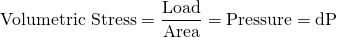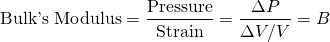Physics - Classical Mechanics - Volumetric Stress and Strain[Image 1]

Introduction

Hey it's a me again @drifter1! Today we continue with Physics and more specifically the branch "Classical Mechanics" to continue with the chapter of Equilibrium and Elasticity. In this article we will get into Volumetric Stress and Strain. So, without further ado, let's dive straight into it!

Volumetric Stress

In the previous article we talked about how forces can affect the length of an object. That so called stress causes an deformation or strain of the object. If the forces acting on an object deform it in such a way that their is a change to the volume of that object, then we are talking about volumetric stress.

Volumetric Stress is equal to the following pressure:Volumetric Strain

When their is volumetric stress, volumetric deformation or volume strain changes the volume of the body. Mathematically, we define that change as:Similar to Tensile Strain, Volumetric Strain also has no units.

Bulk's Modulus of Elasticity

Bulk's Modulus is a numerical constant that describes the elastic properties of a solid or fluid when it is under pressure on all surfaces. The applied pressure reduces the volume of the material.

Mathematically, Bulk's Modulus is defined as:• B = Bulk modulus in N/m2 or Pa
• ΔP = Change of the pressure that applied on the material
• ΔV = Change of the volume of the material
• V = Initial volume of the material
When the value is independent of pressure, this equation is basically a specific form of Hooke's law of elasticity.

Compressibility

The resiprocal of B (1/ B) is called Compressiblity and its S.I. unit is the m2/N or Pa-1.

After the pressure applied is removed the object returns back to its original volume up to a point. The Bulk modulus can be used as a measurement of the ability of a material to withstand changes in volume under compression on all sides. The case where the material can't withstand the pressure or stress is referred to as incompressibility.

RESOURCES:

Images

1.Final words | Next up

And this is actually it for today's post!

Next time we will continue with Isotropic Tension and Strain...

See ya!Keep on drifting!

H2
H3
H4
3 columns
2 columns
1 column